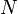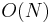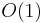The linked list, often referred to simply as a list, is a data structure that organizes a sequence of objects, all of the same type and size, in memory, so that each element except the last is stored along with the address of the next element. Here addresses are not required to occur in arithmetic progression, unlike in arrays. Lists arise in a natural way in the lambda calculus and so they are fundamental to most functional programming languages.

## Implementation

In an imperative programming language, a node of a linked list is typically represented using a record type that contains both the value and a pointer to the next node; the address of the next node can be recovered from the pointer. A sentinel value, such as a null pointer, is often used for the link field of the last node in the list.

Because the list may be empty, we cannot assume that we can remember "where it is" using the address of its first node. Instead, a linked list usually has a head node that contains a pointer to the head of the actual list, which will be null when the list actually contains no first element at all. The head node may also store the length of the list and/or the address of the last element.

### Operations on lists

In the time bounds quoted below,$N$ refers to the length of the list.

• Lists support efficient sequential traversal of their elements, also known as iteration. For example, suppose we wanted to find the sum of all elements in the list. Then we would start at the first element, add its value to an accumulator, follow the link to the next element, and so on until reaching the end of the list, which gives$O(N)$ time.
• An element may be inserted into a list in$O(1)$ time. Suppose we wished to insert node b between nodes a and c. Then we would set the link of b to point to c and set the link of a to point to b (it formerly pointed to c). Contrast this with an array, which requires$O(N)$ time to do the same.
• An element may be deleted from a list in$O(1)$ time; all we have to do is make the link of the previous element point to the next element (this is like "skipping" that element). (A subtlety here, and in a few of the other operations, is that if we are only given the address of the element we wish to delete, we cannot efficiently determine the address of the previous element unless the list is doubly linked; see below.)
• Splicing refers to two operations. Given a list, a starting node, and an ending node within that list, we can easily remove all elements between the starting and ending node from the list, putting them into a separate list; this only requires changing a few links at the boundaries. We may also take a list and insert the entire thing between two adjacent nodes of another list; again, this only requires changing a few links at the boundaries. These operations both take$O(1)$ time.
• Special case: Two lists can be concatenated in$O(1)$ time, if we know in advance the address of the last node of the first.
• Lists do not support efficient random access; if we need to find the 1000th element in a list, we will have to start at the first element and follow 999 links to get to the 1000th. So random access takes$O(N)$ time. If efficient random access is important, it might be better to use an array; if efficient random access and efficient insertion and removal of elements are both important, it is advisable to use a tree.